## LIFE H#RMONIZER

### THE NEXT WAVE IN PERSONAL CAREThe Standard Model of Fundamental Particles

The theories and discoveries of thousands of physicists have resulted in some remarkable insights into the fundamental structure of matter: everything in the Universe found sofar is made from 12 basic fluid-like objects called fundamental particles, spread out as 'ripples' over the continuing space of their 'fields'. They are governed by 4 fundamental forces.

Our best understanding of how these 12 different 'particles' and 3 of the forces are related to each other is encapsulated in the Standard Model of particles and forces.

Developed in the early 1970s, it has successfully explained a host of experimental results and precisely predicted a wide variety of phenomena. Over time and through many experiments by many physicists, the Standard Model has become established as a well-tested physics theory.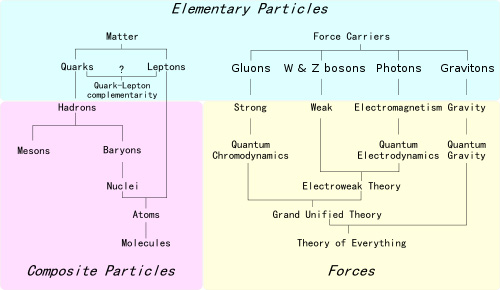12 Matter particles (Fermions)

Everything we see is made of matter particles called fermions, which comprise of: quarks and leptons.

Each group consists of six twin-particles, which are related in pairs, or ‘generations’:

• 1st generation: the lightest and most stable particles,
• 2nd / 3rd generation: heavier and less stable particles.

Quarks:

1st generation: 'up quark' + 'down quark'
2nd generation: 'charm quark' + 'strange quark',
3rd generation: 'top quark' + 'bottom quark'.

Leptons:

1st generation: 'electron' + 'electron-neutrino',
2nd generation: 'muon' + 'muon-neutrino',
3rd generation: 'tau' + 'tau-neutrino'.

The electron, muon, and tau have an electric charge & mass. The ethereal neutrinos are electrically neutral, have minimal mass, and each of the 3 above neutrino 'flavors' propagates through space as a different, constantly changing mixture: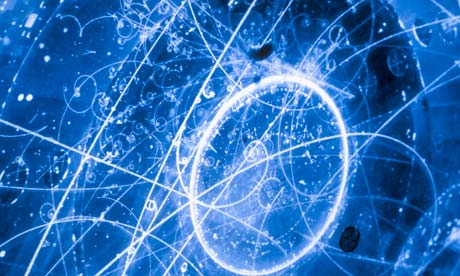Prof. Meyl was the first Physicist to present a Unified Field Theory by calculating the different properties of each of the elementary particles rather than experimentation and then postulation, as has been done by most other Physicists.

He concluded from his extensive research and calculations, that neutrinos are compressed scalar vortices moving faster than the speed of light throughout the entire universe, thus rendering obsolete the idea of dark matter & dark energy.

When neutrinos are moving at the speed of light, they become photons. When a neutrino is slowed to below the speed of light, it becomes an electron.

Neutrinos oscillate between e- and e+. Fusion involves e-, and a lightning flash involves e+. The energy in a vortex acts as a converter of frequency of other particles. The mixture of frequencies is called noise, which can be measured.

Various sterile neutrinos (mass 1 eV) feel only gravity and so fail to interact with ordinary matter at all and, like all the anti-neutrinos, may have non-standard-model interactions.

Prof. Meyl also explains why and how electromagnetic waves (transverse waves) and scalar waves (longitudinal waves) should both be represented in wave equations, as they were when Maxwell presented his equations for the first time.

Transverse electro magnetic waves can be best used for broadcast transmissions like radio and television. Longitudinal scalar waves should be used for private communication systems like cell phones, because they block any 3rd party from wiretapping any conversation between two people.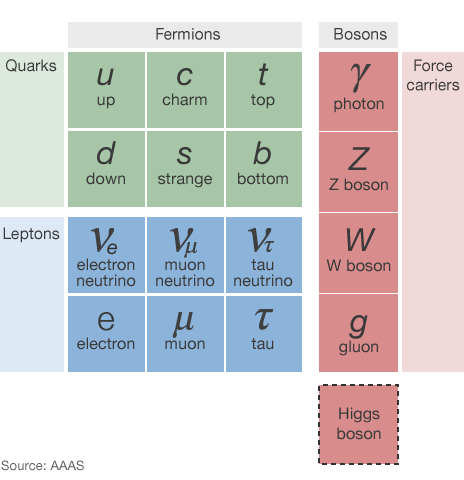4 Forces and carrier particles (Bosons)

There are 4 fundamental forces at work in the Universe. Each works over different ranges and has different strengths:

1. the strong force, strongest of all 4, but only effective over a very short range within a subatomic structure.

2. the weak force, 10 quadrillion times stronger than gravity, weaker than the rest, and only effective over a very short range within a subatomic structure (radioactive decay).

3. the electromagnetic (EM) force (light, electricity), has infinite range but is many times stronger than gravity,

4. the gravitational force, perceived by some a magnetic force, is the weakest but it has an infinite range.

Research has shown that the first 3 of the fundamental forces result from the exchange of force carrier particles, called ‘bosons’. Matter particles transfer discrete amounts of energy by exchanging bosons with each other. Each force has its own corresponding boson particle:

1. the strong force is carried by the ‘gluon’,

2. the electro-weak (EW) force is carried by the ‘W and Z bosons’,

3. the electromagnetic force is carried by the ‘photon’,

4. If it actually exists, the ‘graviton’ should be the corresponding force-carrying particle of gravity.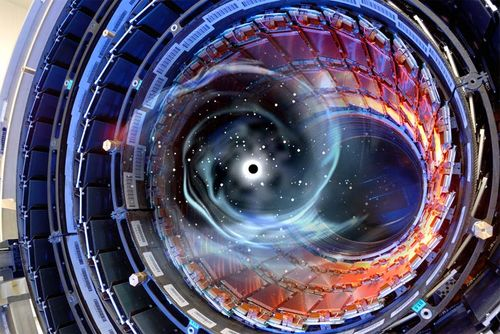The Standard Model includes the electromagnetic, strong and weak forces and all their carrier particles, and explains very well how these forces act on all the matter particles.

Only at the higher energies explored in particle collisions begin the EM and electroweak forces to act on equal terms.

The quantum theory used to describe the micro world, and the general theory of relativity used to describe the macro world. No one has managed to make the two mathematically compatible in the context of the Standard Model.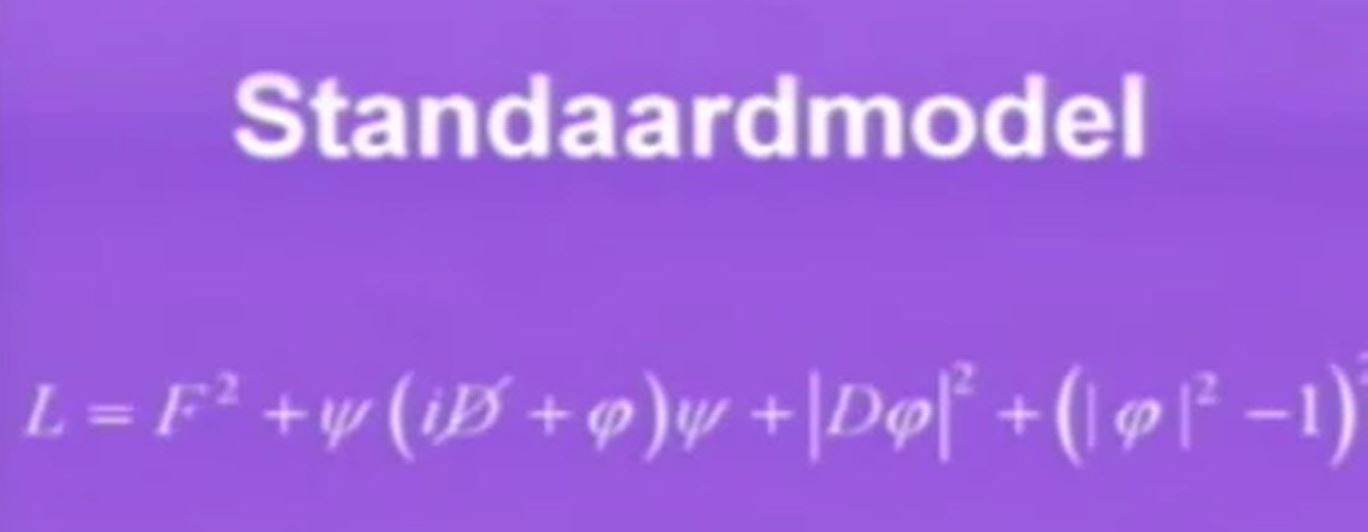However, at the mini scale of particles, the effect of gravity is negligible. Only with matter in bulk, such as in humans or in planets, does the effect of gravity dominate.

Although the Standard Model is currently the best description of the subatomic world, the theory leaves out the questions about why the 4 forces have such differing values, and also about gravity, dark energy, dark matter, and anti-matter: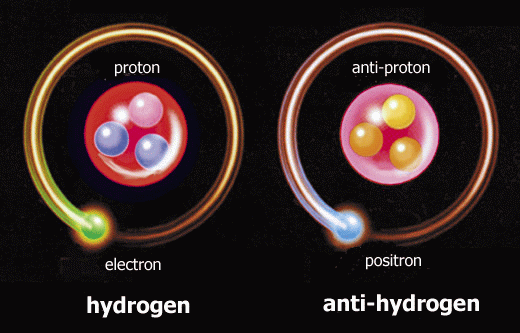Below is a model that reflects all known elementary particles and their interactions. Counting particles is very unreliable because it neglects the interactions between them: particles inside another particle:Click on image for PDF file